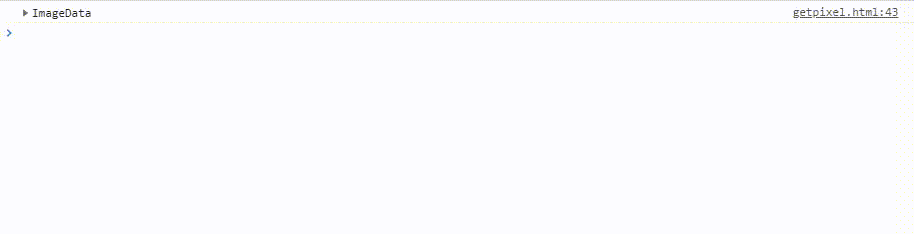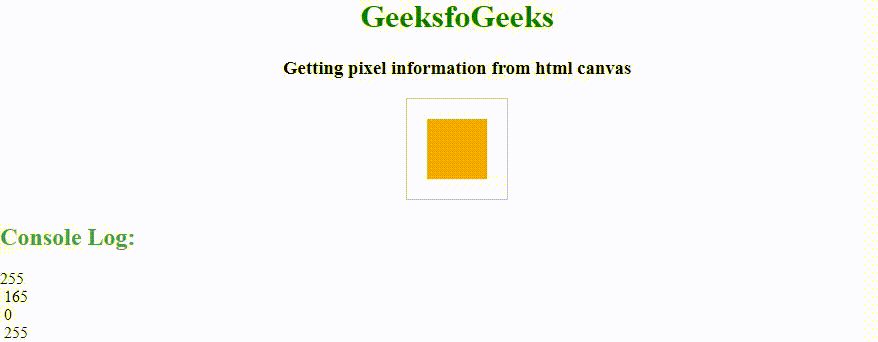# How to get Pixel from HTML Canvas ?

To get the pixel of any specific portion from HTML canvas you can use the HTML canvas getImageData() Method. The getImageData() method usually returns an ImageData object that contains the pixel information of the specified object on the HTML canvas.

Example 1: This example will display each pixel value of the square box. The boxes color is linear gradient so the pixels will change the color value that will change simultaneously.

• Program:

 ` ` `<``html``> ` ` `  `<``head``> ` `    ``<``title``> ` `        ``Getting pixel information ` `        ``from html canvas. ` `    `` ` ` ` ` `  `<``body``> ` `    ``<``center``> ` `        ``<``h1` `style``=``"color:green;"``> ` `            ``GeeksfoGeeks ` `        `` ` `         `  `        ``<``h3``> ` `            ``Getting pixel information ` `            ``from html canvas ` `        `` ` ` `  `        ``<``canvas` `id``=``"GFG"` `width``=``"190"` `height``=``"100"` `                ``style``=``"border:1px solid black;"``> ` `        `` ` ` `  `        ``<``script``> ` `         `  `            ``/* Retrieve canvas with id GFG, ` `                ``and store it in a */ ` `            ``var a = document.getElementById("GFG"); ` ` `  `            ``/* Retrieve a 2D context for the canvas */ ` `            ``var gfg = a.getContext("2d"); ` ` `  ` `  `            ``var geeks =  ` `                ``gfg.createLinearGradient(0, 0, 200, 0); ` `             `  `            ``geeks.addColorStop(0, "green"); ` `            ``geeks.addColorStop(1, "yellow"); ` ` `  `            ``gfg.fillStyle = geeks; ` `            ``gfg.fillRect(20, 20, 150, 150); ` ` `  `            ``/* Define a function find(), that prints ` `            ``the array containing pixel information ` `            ``returned by the getImageData() method */ ` `            ``function find() { ` ` `  `                ``/* Store the pixel information of ` `                ``the canvas at (x,y) coordinate of ` `                ``(20,20) */ ` `                ``var ImageData =  ` `                    ``gfg.getImageData(20, 20, 60, 60); ` ` `  `                ``/* Print the array on console */ ` `                ``console.log(ImageData); ` `            ``} ` `             `  `            ``find(); ` `        `` ` `    `` ` ` ` ` `  ` `

• Output:Example 2: Code for getting color/alpha information of the first pixel. After running the code you can check by changing the color from the line 36.

 ` ` `<``html``> ` ` `  `<``head``> ` `    ``<``title``> ` `        ``Getting pixel information ` `        ``from html canvas. ` `    `` ` ` ` ` `  `<``body``> ` `    ``<``center``> ` `        ``<``h1` `style``=``"color:green;"``> ` `            ``GeeksfoGeeks ` `        `` ` `         `  `        ``<``h3``> ` `            ``Getting pixel information ` `            ``from html canvas ` `        `` ` ` `  `        ``<``canvas` `id``=``"GFG"` `width``=``"100"` `height``=``"100"` `            ``style``=``"border:1px solid black;"``> ` `        `` ` ` `  `        ``<``script``> ` `             `  `            ``// Retrieve canvas with id GFG, ` `            ``// and store it in a  ` `            ``var a = document.getElementById("GFG"); ` ` `  `            ``// Retrieve a 2D context for the canvas ` `            ``var geeks = a.getContext("2d"); ` ` `  `            ``// Set the filling style to red colour ` `            ``geeks.fillStyle = "blue"; ` ` `  `            ``/* Move the cursor to the (x,y) coordinate ` `            ``of (20,20) and then create a rectangle of ` `            ``height and width 60 */ ` `            ``geeks.fillRect(20, 20, 60, 60); ` ` `  `            ``/* Define a function find(), that prints ` `            ``the colour/alpha information of of the ` `            ``first pixel returned by getImageData() ` `            ``method */ ` `            ``function find() { ` `                 `  `                ``var ImageData =  ` `                    ``geeks.getImageData(20, 20, 60, 60); ` ` `  `                ``/* Stores the red color information of  ` `                ``the first pixel */ ` `                ``red = ImageData.data; ` ` `  `                ``/* Stores the green color information of  ` `                ``the first pixel */ ` `                ``green = ImageData.data; ` ` `  `                ``/* Stores the blue color information of  ` `                ``the first pixel */ ` `                ``blue = ImageData.data; ` ` `  `                ``/* Stores the opacity of the first pixel */ ` `                ``alpha = ImageData.data; ` `                ``console.log(red); ` `                ``console.log(green); ` `                ``console.log(blue); ` `                ``console.log(alpha); ` `            ``} ` `            ``find(); ` `        `` ` `    `` ` ` ` ` `  `      `

Output:My Personal Notes arrow_drop_upCheck out this Author's contributed articles.

If you like GeeksforGeeks and would like to contribute, you can also write an article using contribute.geeksforgeeks.org or mail your article to contribute@geeksforgeeks.org. See your article appearing on the GeeksforGeeks main page and help other Geeks.

Please Improve this article if you find anything incorrect by clicking on the "Improve Article" button below.